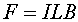Magnetic fields and forces

A particle of charge q moving with velocity v through a magnetic field B feels a forcewhere q is the angle between the direction of the magnetic field and the direction of the velocity. The direction of the force is perpendicular to both the velocity and magnetic field: see the right-hand-rule page. If a particle moves parallel to the magnetic field, it feels no force.

The magnetic field is given in units of Tesla, where 1.0 T = 1.0 N·s/(mC). A magnetic field on the order of a Tesla is very strong, and can only be created by rather expensive magnets. The magnets you stick to a refrigerator produce fields on the order of 10-3 T.

A wire carrying an electric current has moving charge and therefore feels a force in a magnetic field. If a segment of wire of length L has N charges of size q moving with velocity v perpendicular to the field, the force is F = N q v B. The current is the amount of charge moving past a given point per unit time, I = N q v / L . Putting the last two equations together yieldsThus the force per unit length felt by a segment of wire in a magnetic field is the current times the magnetic field. Like the original force equation, this also needs a factor of sin q if the field and the wire are not perpendicular to each other.

Examples       Magnetic fields and forces index        Lecture index